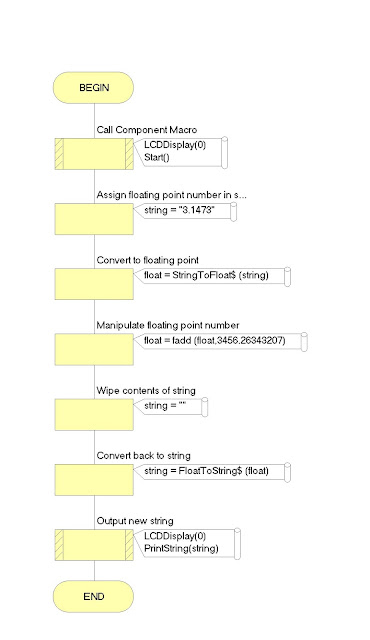## Saturday, 26 December 2015

### Geometric Progression - Write A Program To Find SUM of GP Sequence -C Programming Examples

As we have started C Programming coding with Example Series, We have published last post Write A Program To Find SUM of AP. We have chosen to start with mathematical series and in this post you will find some basic idea and some useful concepts about Geometric Progression (G.P.).You can download C Codes below.Please let us know your feedback.

Integrated Environment : TURBO C/C++ - Getting Started
TURBO C/C++: Installer and Installation
C Language Programming With Examples-INDEX

Arithmetic Progression Step-wise Video Tutorial(See C- Codes Below ):

Geometric Progression Basic Guide:-A sequence of non-zero numbers is a Geometric Progression (G.P.) if the ratio of any term and its preceding term is always constant.

A Geometric Progression (G.P.) is given by a, ar, ar2, ar3, ...
where a = the first term , r = the common ratio

Examples for Geometric Progressions
1, 3, 9, 27, ... is a geometric progression (G.P.) with a = 1 and r = 3

2, 4, 8, 16, ... is a geometric progression (G.P.) with a = 2 and r = 2

Nth term of a geometric progression (G.P.)

Sum of first N terms in a geometric progression (G.P.)

## Wednesday, 23 December 2015

### Arithmetic Progression - Write A Program To Find SUM of AP C Programming Examples

We re starting C Programming Tutorial Series.We will try to satisfy our readers by giving some quality content in this series.

To Begin-with we have chosen to start with mathematical series.First series that always troubles students is Arithmetic progression.In this post you will find some basic idea and some useful concepts below.

Arithmetic Progression Step-wise Video Tutorial(See C- Codes Below ):

Integrated Environment : TURBO C/C++ - Getting Started
TURBO C/C++: Installer and Installation
C Programming Basic Questions
Variables-Pointer-Array-Files
Write A Program To Find SUM of Geometric Progression
Write A Program To Find SUM of Infinite Harmonic Progression

Arithmetic Progression / Sequence  Mathematically Explained:

Arithmetic progression (AP) is a sequence of numbers in which each term after the first is obtained by adding a constant, d to the preceding term. The constant d is called common difference.

An arithmetic progression is given by a, (a + d), (a + 2d), (a + 3d), ...
where a = the first term , d = the common difference

Sum of first N Terms of AP:

Nth term of an arithmetic progression Tn = a + (n – 1)d
(where Tn = nth term, a= the first term , d= common difference)

Number of terms of an arithmetic progression
n=(l−a)d+1
(where n = number of terms, a= the first term , l = last term, d= common difference)

## Tuesday, 15 December 2015

### String To Float Data Type Conversion Using Microchip Controller PIC16F877A In Flowcode Programming SoftwareIn previous post Float to String Conversion we have seen how to convert Float types of data in String type of Arrays using simple programming techniques.In this project we will be using FlowCode Software for writing a program to convert String Array or Array of Character to Floating type of Data using micro-controller of Microchip company PIC16 Family Micro controller PIC16F877A for testing and Simulation.This Project shows how we are outputting results values to LCD ( Liquid Crystal Display ).Varying the delay and write-erase sequence can be manipulated to different types of combination and lots of desired result.
Flow code program Snapshot(Above).

Here value used is of PI=3.1473

Command used is
string = FloatToString\$ (float)
float = StringToFloat\$ (string)

Recent Related Programs
Keypad and LED Interfacing With PIC16F88

Here is the complete list of projects:
Tutorials for Flow Code Programming and Simulation

FlowCode Simulation [How To Do] Video Tutorial:

A brief introduction to the concept of Data types and String Array:-

Data types in c refer to an extensive system used for declaring variables or functions of different types. The type of a variable determines how much space it occupies in storage and how the bit pattern stored is interpreted.